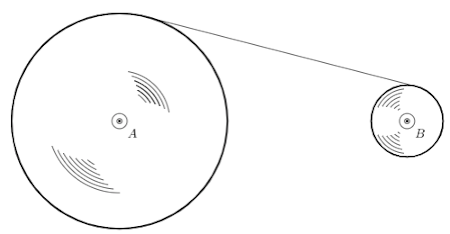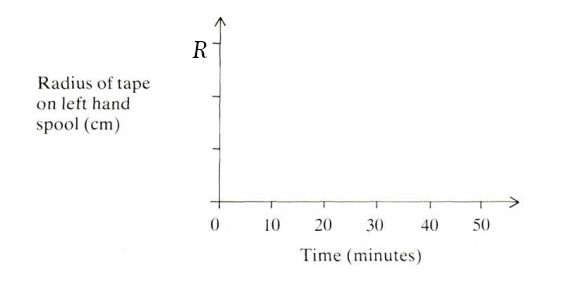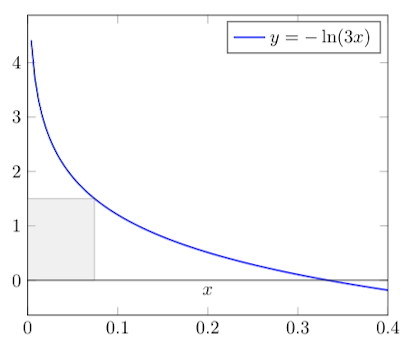MT #4: Limits and derivatives

Instructions

• You are responsible for keeping time. Email all your solutions by 20:00 Hrs IST.
• Find an email from me with the subject line ‘Mock test 4: Limits and derivatives’. Send your solutions (images) as replies to this email.
• Adjust/Reduce the resolution of the camera so that each image is less than 500 KB in size.
• Total marks: 100 (10x4=40 for Part A + 6x10=60 for Part B)

For students who miss the live test (members only)
Self-administer the mock test and email your solutions before 11 Mar, 23:59 Hrs. Your solutions will be evaluated but marks won’t be counted for official use in the future. Solutions submitted after 11 Mar, 23:59 Hrs will not be evaluated. As per the rules of CMI entrance exam, no calculators or log tables must be used.

Submission file: Write answers to all the ten questions on a single sheet of paper. Email a picture of your answer sheet. Name the file as PartA.jpg.

For this part, answers must be written without any explanation.

1. Suppose $$p(x)$$ and $$q(x)$$ are polynomials such that: $p(x) = (x-1)q(x)$ If $$p^{\prime}(1)=1$$, what is the value of $$q(1)$$?

2. Let $$D_1$$ denote the set of positive one-digit numbers, $$D_3$$ the set of three digit numbers and in general $$D_n$$ the set of $$n$$-digit numbers. Consider the following sum for any odd $$n$$: $S_{n} = \sum_{k_1\in D_1} \frac{1}{k_1} + \sum_{k_3\in D_3} \frac{1}{k_3} + \sum_{k_5\in D_5} \frac{1}{k_5} + \cdots + \sum_{k_n\in D_n} \frac{1}{k_n}$ As $$n$$ tends to infinity, to what value does $$S_n$$ tend to?

3. Consider the function $$f: \mathbb{R} \rightarrow \mathbb{R}$$ given by $$f(x)=(x-1)|(x-1)(x-2)|$$. The function $$f$$ is
1. differentiable at $$x=1$$ and $$x=2$$.
2. differentiable at $$x=1$$ but not at $$x=2$$.
3. differentiable at $$x=2$$ but not at $$x=1$$.
4. neither differentiable at $$x=1$$ nor at $$x=2$$.
Pick the right option.

4. What is the smallest value of $$n$$ for which the following limit exists? $\lim_{x \rightarrow 0} \frac{\sin^{n}x}{\cos^{4}x(1-\cos x)^{3}}$

5. We have a tape that is wound as a spool around axle $$A$$. There is an axle $$B$$ that winds the tape around itself at constant speed. In other words, a fixed length of tape is transfered in one minute from spool $$A$$ to $$B$$. Let the instantaneous radius of the spool $$A$$ be $$r$$. Intially, at $$t=0$$ minutes, $$r=R$$ cm. At $$t=50$$ minutes, $$r=0$$ cm. Qualitatively, sketch the value of $$r$$ as a function of time.Sketch the value of $$r$$ against time.6. Let $$f_{0}(x)=(\sqrt{e})^{x}$$. For $$n\geq 0$$, we define $$f_{n+1}(x)=f_{n}^{\prime}(x)$$. Compute $$\sum_{i=0}^{\infty} f_{i}(1)$$.

7. Alice and Bob are standing on a playground and Alice is 400m directly East of Bob. Alice starts to walk North at a rate of 3 m/sec, while Bob starts to walk South at the same time at a rate of 7 m/sec. After 30 seconds, at what rate is the distance between Alice and Bob changing?

8. Suppose $$p(x) = x^3 + x^2 + 2x + k$$. Which of the following statements is true?
1. $$p(x)$$ has exactly one real root for any value of $$k$$.
2. For some real number $$k$$, $$p(x)$$ has more than one real root.

9. Calculate the value of the infinite series: $\sum_{k=1}^{\infty} \frac{1}{2 k^{2}-k}$

10. Evaluate: $\lim_{n \rightarrow \infty} 4^{n}\left(1-\cos \left(\pi / 2^{n+1}\right) \right)$

Part B: Subjective questions

Submission files: Each question in this part must be answered on a page of its own. Name the files as B1.jpg, B2.jpg, etc. In case you have multiple files for the same question, say B4, name the corresponding files as B4-1.jpg, B4-2.jpg, etc.

Clearly explain your entire reasoning. No credit will be given without reasoning. Partial solutions may get partial credit.

• B1. Consider an axis-parallel rectangle with its bottom-left corner at the origin and top-right corner on the curve $$y=-\ln 3x$$. What is the maximum area that can be attained by any such rectangle?B2. A fractal region is constructed as follows. Initially, a unit square is added at $$t=1 s$$. At each subsequent time step $$t$$, new squares of size $$s_t$$ are added to the region as follows.

For each square $$S$$ that was added at time step $$t-1$$:

• Add a square with side length $$s_t = s_{t-1}/2$$ to each of the free corners of $$S$$.
The figure below shows the region after the third time step. Squares of the same shade were added at the same time step. As $$t$$ tends to infinity, what value does the area of the region approach?B3. Let $$x_{0}=1$$ and $x_{n}=\frac{3+2 x_{n-1}}{3+x_{n-1}}$ for $$n=1,2, \ldots .$$
(a) Prove that the sequence converges, that is, $$\lim_{n \rightarrow \infty} x_{n}$$ exists. [6 marks]
(b) Find the value of the above limit. [4 marks]

B4. Find the value of the limit: $\lim_{x \rightarrow 0} \frac{1}{x} \ln \left(\frac{e^{x}-1}{x}\right)$

B5. A monic polynomial is a polynomial with leading coefficient as 1. Suppose $$p$$ is a monic polynomial with real coefficients. Let $$\lim_{x \rightarrow \infty} p^{\prime \prime}(x)=\lim_{x \rightarrow \infty} p\left(\frac{1}{x}\right)$$ and $$p(x) \geq p(2)$$ for all $$x \in \mathbb{R}$$. Find $$p(x)$$.

B6. Let $$p(x)$$ be a polynomial with only real roots. Show that for every $$x$$: $p^{\prime}(x)^2 \geq p(x) p^{\prime\prime}(x)$